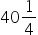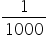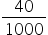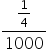Mathematics
Easy

Question

# Convertmg into grams.

## The correct answer is: 0.04025

### We know,1000mg = 1g Or 1mg =gThen,mg =+= 0.040 + 0.00025= 0.040251 mm=m = 2000 mm = 2000 ÷ 1000 = 2m

One g is equal to 1000 mg. therefore, (4 x 40 +1)/4 mg = 161/4 mg= 161/4  x 1/1000 g = 0.4025 g

### Related Questions to study#### With Turito Foundation.#### Get an Expert Advice From Turito.